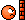# A question in double titration

Gold Member
2020 Award

## Homework Statement

:[/B]

A mixed solution of KOH and sodium carbonate required 15ml of (N/20) HCl solution when titrated with phenolphthalein indicator. But the same amount of solution, when titrated with methyl orange as an indicator, required 25ml of the same HCl. The amount of KOH in the solution is:

1. 0.014g
2. 0.14g
3. 0.028g
4. 1.4g

:[/B]

## The Attempt at a Solution

:[/B]

Let the number of equivalents of HCl be ##x## and that of sodium carbonate be ##y##.

So, using phenolphthalein, $$x+y = \frac {15}{1000} × \frac {1}{20}$$
Using methyl orange,

$$x+2y = \frac {25}{1000} × \frac {1}{20}$$
Solving these two equations, I get a value for ##x## with which I'm not getting any option for the weight of KOH. Where am I going wrong?

Borek
Mentor

Or, if you are sure about it, show what you got.

•Wrichik Basu
Gold Member
2020 Award

Or, if you are sure about it, show what you got.
Concept is OK?

Gold Member
2020 Award

Or, if you are sure about it, show what you got.
Subtracting first equation from second, $$y=1.25×10^{-3} \; - 0.75×10^{-3}$$ $$Or, \quad y=0.5×10^{-3}$$.
So, $$x=0.25×10^{-3}$$
Therefore, weight of KOH=0.014g.

Very sorry, I mistakenly put the weight of NaOH instead of KOH.Thank you.

Borek
Mentor
Happens to everyone.

•Wrichik Basu